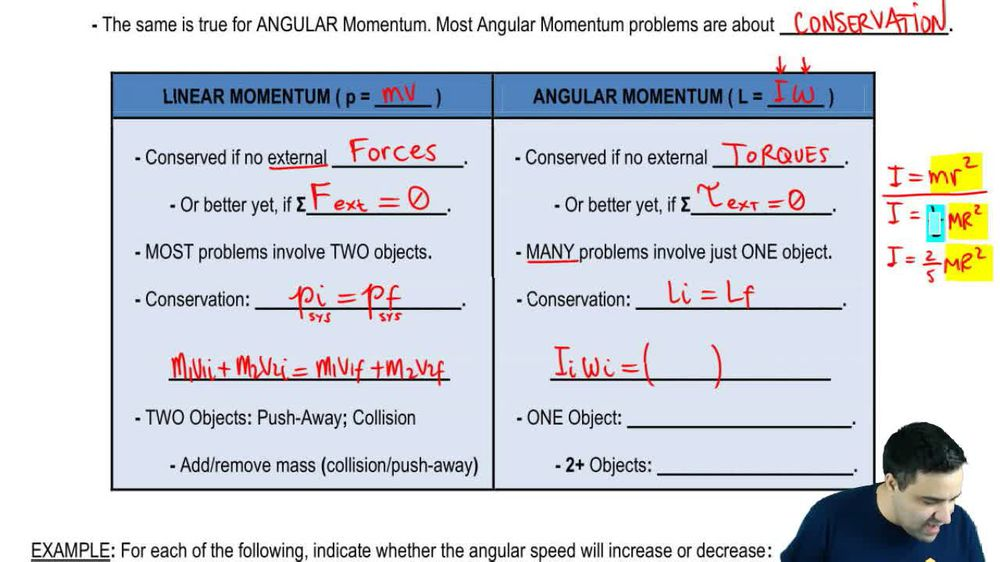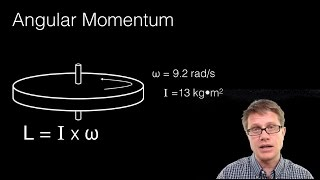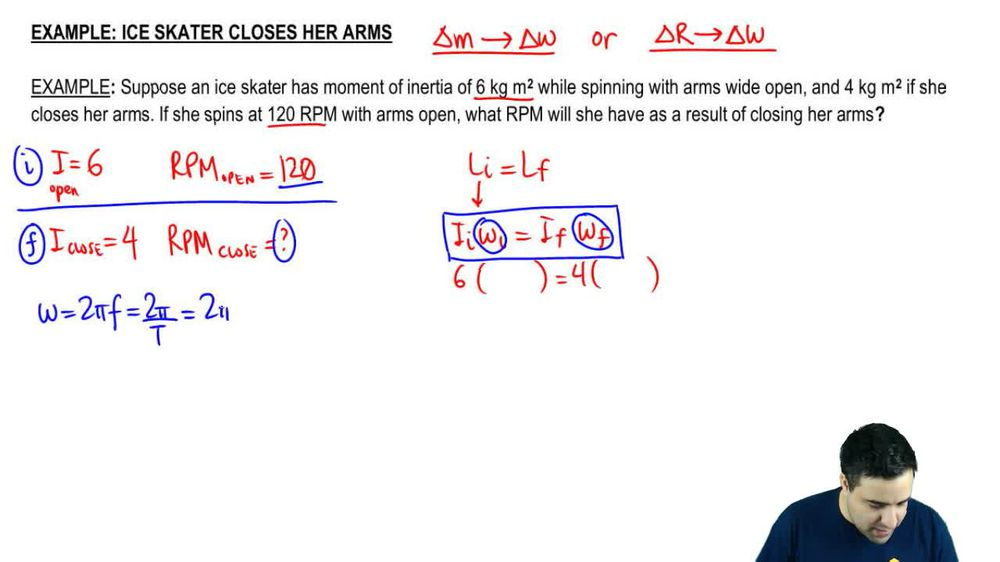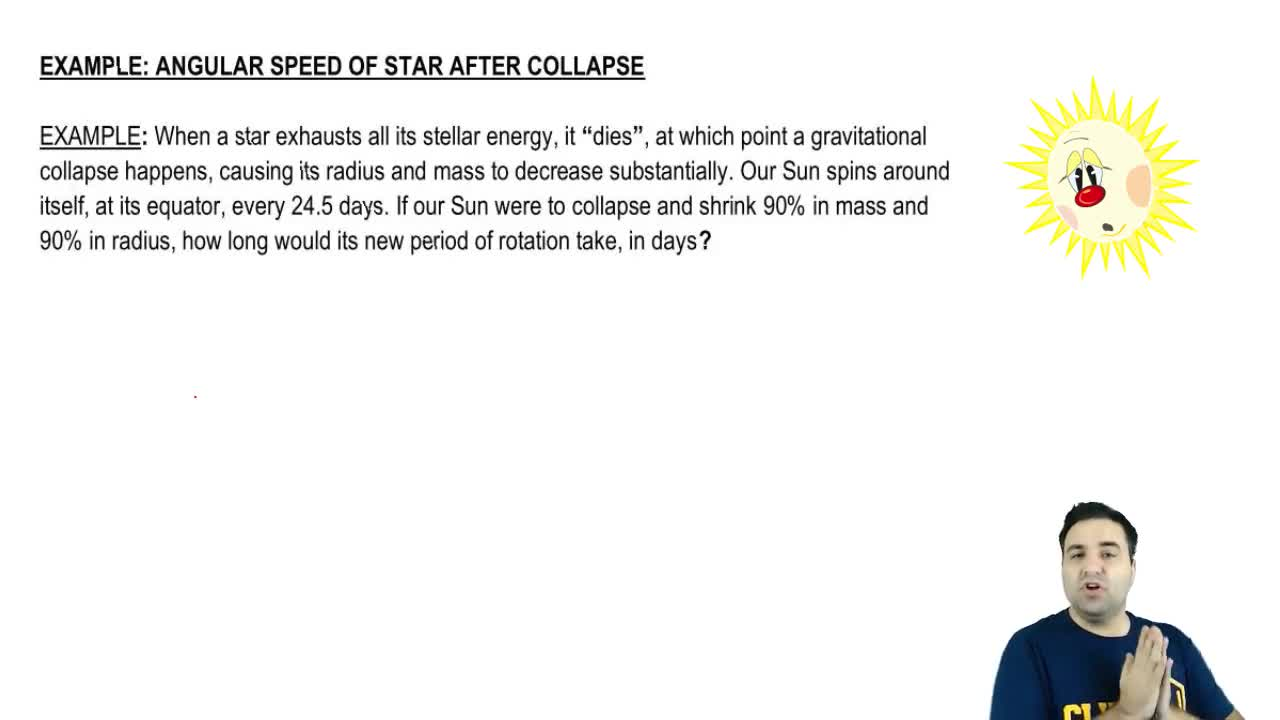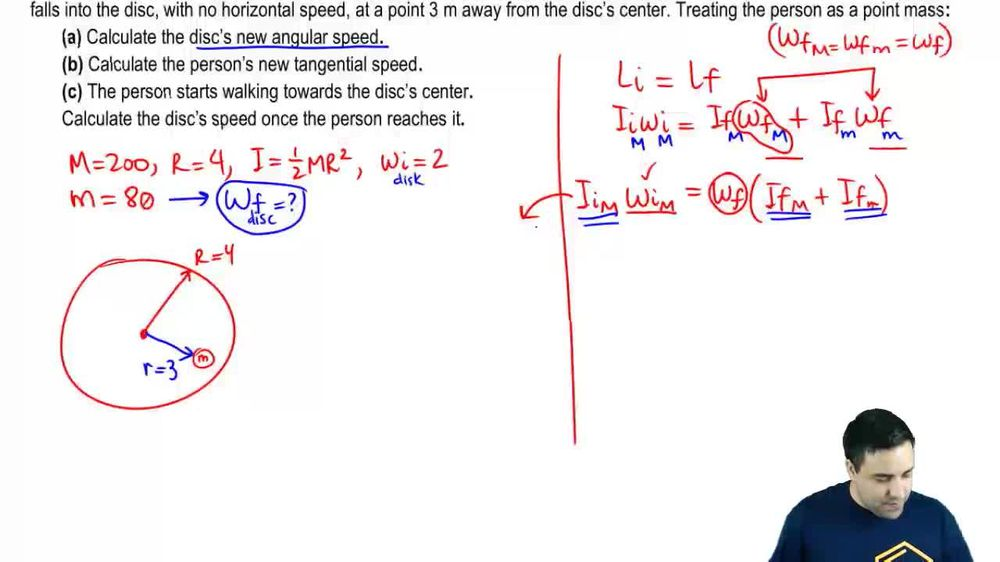Start typing, then use the up and down arrows to select an option from the list.
1. 16. Angular Momentum2. Conservation of Angular Momentum# Ice skater closes her arms

by Patrick Ford
100 views
2
0
Hey, guys. So let's check out this classic example of conservation of angular momentum. Okay? Remember, conservation of angular momentum are about objects that are spinning that will change either their m and then that will cause a change in omega. Or they will change the radius since of some sort. And that will cause a change in omega. Those are the two types that you have to look out for. So here we have a nice skater that has a moment of inertia of six. So I equals six when she spins with her arms open. So her eye is six. When she spins with arms will open. And four, if she closes her arm, I close equals form. It says here if she spins with six with rpm with their arms open. So rpm open is 20. What? Our pm you should have is a result of closing her arms. What will be our PM close? Okay, so you can think of open as in this show, because that's where we start. And you can think of clothes as final. Okay, so we're gonna use the conservation of angular momentum equation which is l I equals L f. In this case, we have one person. So it's just gonna be this is gonna be just Iomega for one person. I initial or make initial equals I final Omega final. Okay, the eyes were given, three eyes were given, so this is going to be initial is six, and then I have a mega. I'm gonna make a little space here. And then this is four and I'm gonna take a little space here. Now, notice that this is Omega and this is Omega. But I gave you one rpm and I asked you for another rpm. So this whole question is in terms of RPM, but the equations in terms of omega, like, usual, all of our equations are in terms of Omega. We always have to convert rpm into omega. But what I want to show you is that you can actually think of You can actually rewrite this equation here in terms of rpm, so let's do that real quick. Remember, um, Omega is two pi f or two pi over tea or two pi. I'm gonna plug in f here, and it's gonna be rpm over 60. So what I don't wanna do real quick cause I want to show you that there are three variations of this question. OK, so let's do that real quick. So this is like the official legit version. Number one. Here's version number two instead of omega. I'm gonna write two pf and look what happens. I initial two pi frequency initial equals I Final two pi frequency final notice that the two pies cancel and you end up with I f equals I f Okay, so this is another version of this equation. You can just basically replace Omega by frequency, okay? And they are both on the top. If you do this with period, this is what you get. This is version two I two pi period. Initial equals I Final Two Pi period final. Okay, these guys will cancel and you end up with I initial period initial because I final period final and you could do the same thing for our PM. Okay? And this is the last one. That's the one we're gonna use here. You weaken. Say I in issue now instead, off instead of two pf, we're gonna use two pi r p m over 60 So two pi our PM over 60 equals. I final trying to squeeze us in here. Out of the way two pi our PM over 60. Okay. And almost unfit. And look what I can do here. I can cancel the two pies in the in the 60 and you're left with I initial rpm initial equals I final rpm final. Okay, so this is the conservation equation. But you can think of it in these three alternative versions as well. This just makes it really easy for you to solve these questions, Uh, by basically briefly rewriting the equation. So one point I wanna make here is that a way to know how to make thes thes exchanges very quickly is Look at Omega. Omega is on the top. It's on the denominator. A numerator up here f is in the numerator appear they're both up top. That's why they both show up up top here. T is on the denominator. That's why T shows up at the bottom when you replace it and rpm is at the top. That's why rpm shows up at the top here. Okay, so in this question, were actually we don't have to convert the RPMs into omega and then back into our PM, we can just actually plug in the rpm, so I'm gonna plug in rpm initial rpm final. Okay? It would have been quick to just replace stuff, but I wanted to show you that we can do this. So rpm in issue is rpm initial is open, which is 1 20. And then this is four rpm final. So rpm final will be six times 1 divided by four. Okay. And the answer here is 180 our PM Now, the last point I wanna make. So this is the final answer. Boom. The last point I wanna make is notice that our I went from 6 to 4. It is it changed by a factor of 1.5. It went down by a factor of 1.5 and then So it went down by 1.5. Um, and then the rpm went from 1 20 to 1 80. It went up by a factor of 1.5. And that's because conservation momentum here of angel momentum is a linear relationship. There's no squares or whatever. Eso if one goes down by by 1.5. The other one has to go up by 1.5. Okay, Alright, so that's it for this one. This question is actually really easy. I just took a little longer because I wanted Thio do a little bit of analysis and I wanted to introduce you to these three alternative versions of the conservation equations so you can solve some of these questions faster. All right, so that's it for this one. Let me know if you need any help. If you have any questions and let's keep going.